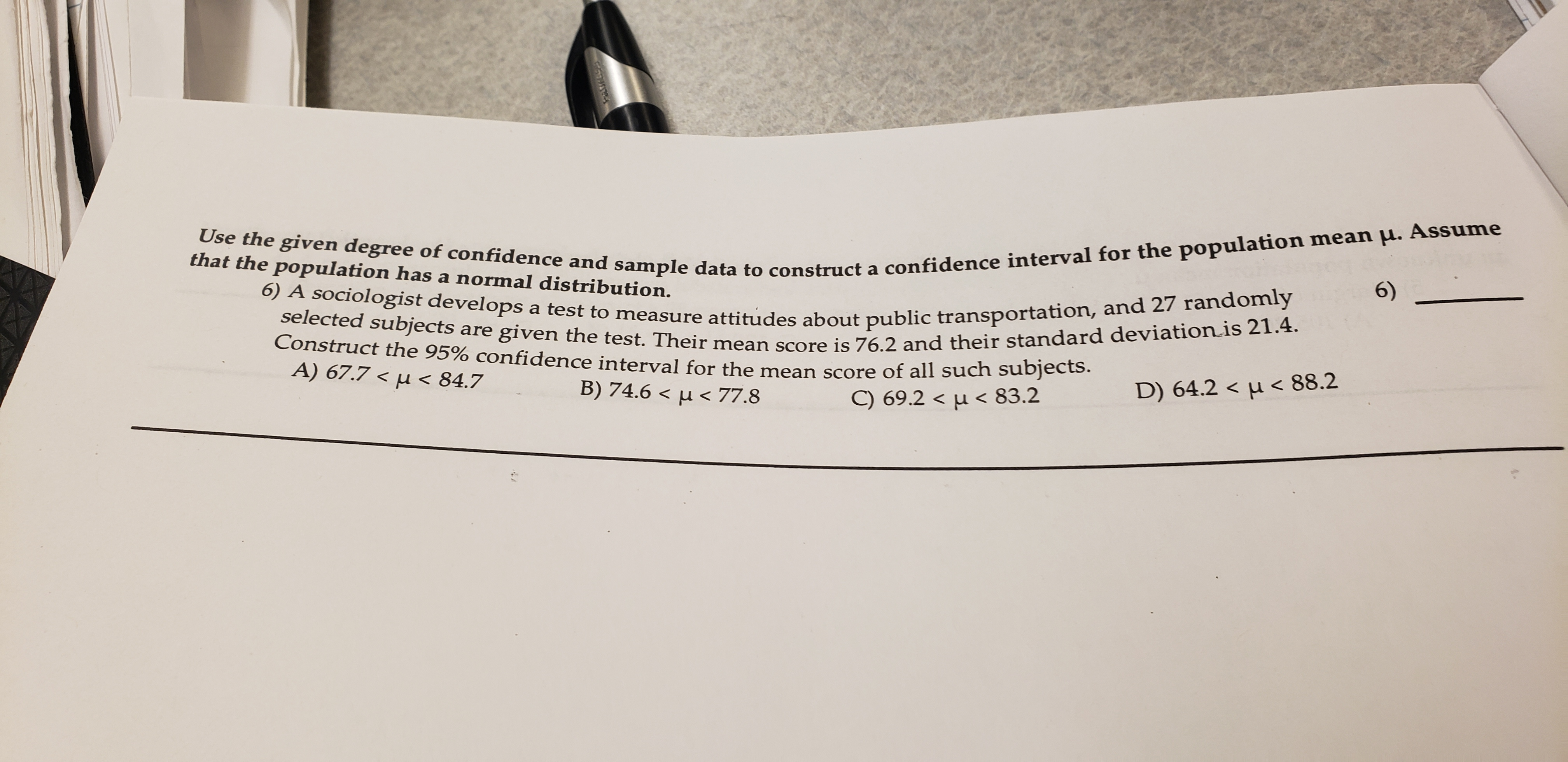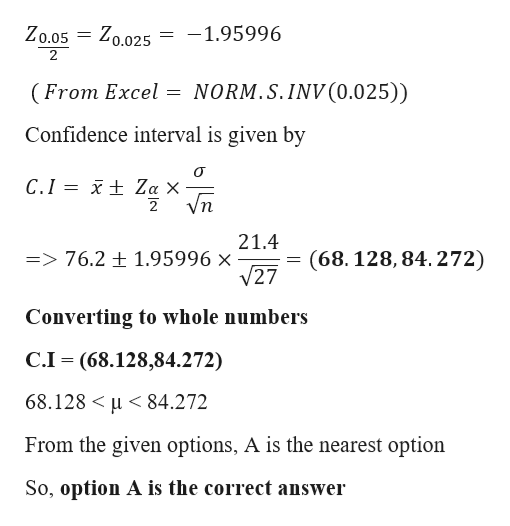# TaressAssumeUse the given degree of confidence and sample data to construct a confidence interval for the population mean u..that the population has a normal distribution.6)6) A sociologist develops a test to measure attitudes about public transportation, and 27 randomlyselected subjects are given the test. Their mean score is 76.2 and their standard deviation is 21.4.Construct the 95% confidence interval for the mean score of all such subjects.A) 67.7 < u < 84.7B) 74.6 < u< 77.8C) 69.2 < u< 83.2D) 64.2 < u

Question
35 viewshelp_outlineImage TranscriptioncloseTaress Assume Use the given degree of confidence and sample data to construct a confidence interval for the population mean u.. that the population has a normal distribution. 6) 6) A sociologist develops a test to measure attitudes about public transportation, and 27 randomly selected subjects are given the test. Their mean score is 76.2 and their standard deviation is 21.4. Construct the 95% confidence interval for the mean score of all such subjects. A) 67.7 < u < 84.7 B) 74.6 < u< 77.8 C) 69.2 < u< 83.2 D) 64.2 < u<88.2 fullscreen
check_circle

Step 1

Given data

Sample size(n) = 27

Sample mean = 76.2

Population standard deviatio...help_outlineImage TranscriptioncloseZo.025 -1.95996 Zo.05 (From Excel NORM.S.INV(0.025)) Confidence interval is given by xt Za X Vn C.I 21.4 1.95996 x V27 (68.128, 84. 272) => 76.2 Converting to whole numbers C.I (68.128,84.272) 68.128 84.272 From the given options, A is the nearest option So, option A is the correct answer fullscreen

### Want to see the full answer?

See Solution

#### Want to see this answer and more?

Solutions are written by subject experts who are available 24/7. Questions are typically answered within 1 hour.*

See Solution
*Response times may vary by subject and question.
Tagged in

### Statistics